﻿ Evaluation of Energy Losses in Pipes

### Evaluation of Energy Losses in Pipes

LAHIOUEL Yasmina, LAHIOUEL Rachid

American Journal of Mechanical Engineering

## Evaluation of Energy Losses in Pipes

LAHIOUEL Yasmina1,, LAHIOUEL Rachid2

1Laboratoire d'Analyse Industrielle et de Génie des Matériaux (LAIGM), Faculté des sciences et de la Technologie, Université de Guelma, B.P. 401, Guelma

2Laboratoire de Physique de Guelma (GPL), Université de Guelma, B.P. 401, Guelma, Algérie

### Abstract

Energy losses in pipes used for the transportation of fluids (water, petroleum, gas, etc.) are essentially due to friction, as well as to the diverse singularities encountered. These losses are usually converted into head reductions in the direction of the flow. The knowledge of data of such transformation allows the determination of the necessary power needed for the transportation of the fluid between two points. It constitutes the necessary calculation basis necessary for the design and analysis of transport and distribution networks. The review of the different relationships allowing the determination of these losses and their comparison to the experimental results obtained by the authors constitute the object of this study.

• LAHIOUEL Yasmina, LAHIOUEL Rachid. Evaluation of Energy Losses in Pipes. American Journal of Mechanical Engineering. Vol. 3, No. 3A, 2015, pp 32-37. http://pubs.sciepub.com/ajme/3/3A/6
• Yasmina, LAHIOUEL, and LAHIOUEL Rachid. "Evaluation of Energy Losses in Pipes." American Journal of Mechanical Engineering 3.3A (2015): 32-37.
• Yasmina, L. , & Rachid, L. (2015). Evaluation of Energy Losses in Pipes. American Journal of Mechanical Engineering, 3(3A), 32-37.
• Yasmina, LAHIOUEL, and LAHIOUEL Rachid. "Evaluation of Energy Losses in Pipes." American Journal of Mechanical Engineering 3, no. 3A (2015): 32-37.

 Import into BibTeX Import into EndNote Import into RefMan Import into RefWorks

12
Prev Next

### 1. Introduction

Pipe technology is based on the universal principles of fluid flow. When a real (viscous) fluid flows through a pipe, part of its energy is spent through maintaining the flow. Due to internal friction and turbulence, this energy is converted into thermal energy. Such a conversion leads to the expression of the energy loss in terms of the fluid height termed as the head loss and usually classified into two categories. Essentially due to friction, the first type is called linear or major head loss. It is present throughout the length of the pipe. The second category called minor or singular head loss is due to the minor appurtenances and accessories present in a pipe network. The appurtenance encountered by the fluid flow which is a sudden or gradual change of the boundaries results in a change in magnitude, direction or distribution of the velocity of the flow. This classification into major and minor head losses is rather relative. For a pipeline of small length having many minor appurtenances, the total minor head loss can be greater than the frictional head loss. In petroleum and water distribution networks, the pipelines are of considerable length and therefore the terms major head loss and minor head loss can be used without any confusion.

A great number of studies were carried out in order to achieve a general and precise formulation of the diverse types of head losses.  was the first to have come out with a relation for the head loss. As brought up by , Darcy contributed greatly to the application of the derived relation, thus associating his name with that of Weisbach. The relation is therefore most commonly known as the Darcy-Weisbach formula. It essentially depends on the friction coefficient and the relative roughness.

The friction coefficient is a function of the flow regime characterised by the Reynolds number. Several explicit and implicit relationships were proposed for the friction coefficient.  performed extensive experimentations involving smooth and artificially roughened pipes achieved using sand particles of uniform size. The Nikuradse diagram also known as the Stanton diagram or the Stanton-Pannel diagram, is the result of these investigations. Comparing the results included in Nikuradse’s diagram,  found that its curves do not match with those of actual pipes. However, by introducing the concept of equivalent surface roughness, it is possible to use Nikuradse’s results for commercial pipes.

Several other investigators provided the literature with diverse diagrams.  presented a diagram for commercial pipes using several non-dimensional groups.  plotted the friction coefficient represented by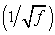against the Karman number represented by: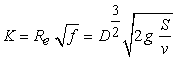. He produced the necessary curves for the Colebrook transition zone. L. F. Moody suggested to Rouse converting his diagram by plotting the friction coefficient against the Reynolds number which he refused.  then plotted it himself, producing the actual universally known Moody diagram allowing the determination of the friction coefficient as a function of the Reynolds number and the ratio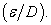Other investigators provided the literature with relationships.  suggested a relationship between the friction coefficient and the Reynolds number applicable solely for smooth turbulent flows. For the same regime,  suggested a relationship similar to that of Blasius. For a transitional turbulent flow,  derived a relationship which is presently commonly known as the Colebrook-White equation. For a rough turbulent flow, Prandtl  suggested a relationship expressing the friction coefficient as a function of the ratio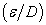. It is now widely known as the Karman-Prandtl equation.

All these relationships are implicit implying the use of a try-and-error procedure in order to achieve a solution leading to a value of the friction coefficient. Explicit relations expressing the friction coefficient for all regimes of flow are available. For a smooth turbulent flow,  suggested a simple explicit relation based on the Karman-Prandtl equation while  proposed a simpler one. For a transitional turbulent flow,  enriched the specialised literature with a highly accurate relationship, on which the relations of [1, 5, 10, 13, 23, 29, 30] linking the friction coefficient f to the Reynolds number Re and the relative pipe roughnesshave been based and led to an appreciable accuracy.

Relationships applicable for all regimes are also available.  proposed a friction factor equation which should be applicable for all fluid flow regimes whereas  derived explicit solutions for the Prandtl and Colebrook-White equations. One of the widely used formulas used is that developed by . It expresses the head loss as a function of diameter, flow rate and length using an empirical coefficient. The Hazen-Williams coefficient as it has since been known depends on the pipe material and the flow velocity. Values of the Hazen-Williams coefficient applicable for diverse common pipe materials are recommended by  who tabled them after performing a great amount of experimentations. The Hazen-Williams formula was a subject of interest for many investigators. Based on his own tests,  confirmed it while [Janna et al, 1978] tried to modify it by introducing a new coefficient which vary with the Reynolds number, the relative roughness and the flow velocity. Earlier in 1923, as reported by  Strickler had suggested a simpler relationship based on a fixed coefficient. Later on, other empirical relationships were proposed. Hence,  suggested his relationship as early as 1930. It is similar to that of Hazen-Williams except for the use of a fixed coefficient. In 1965 as reported by , Calmon and Lechapt, suggested a relationship including three coefficients which vary with the pipe roughness. The Manning formula known as such because it has firstly been derived by Manning in 1891  has, as reported by  and , since received a lot of contributions and is still being used.

The various experimentations carried out by the authors in order to determine the linear and singular head losses taking place in a variety of pipes of different dimensions and roughness are presented. Water is used at different flow regimes. The obtained linear results are compared to those computed using the most common pipe flow formulas presented earlier (Darcy-Weisback, Hazen-Williams, Manning, Strickler, Scobey and Calmon & Lechapt) leading to the domain of application and accuracy of these relationships.

Eight singularities widely used in distribution networks have also been investigated. They include sudden enlargements and contractions, 45° and 90° bends of different radiuses of curvature, Venturi meters, orifice meters, gate valves and ball valves. The results obtained are compared to those available in the literature.

### 2. Experimental Method

The experimental investigations were carried out on a test bench mainly constituted by a hydraulic bench providing the necessary flow discharge, a network of pipes of different dimensions and roughness able to simulate both frictional (major) and singular (minor) head losses.

The hydraulic bench used to distribute the water at the required flow rates, is principally constituted by a reservoir and two pumps which may be used in series or in parallel . The water is pumped from reservoir to the pipes network through a closed circuit. A regulation valve, a direct rotameter, and water and mercury manometers are used to regulate the fluid flow and to determine the pressure in terms of head difference respectively.

The network is constituted by PVC pipes of different diameters and roughness. Four are pipes used for frictional head losses. Their diameter vary from 13 mm to 25 mm, and their surface roughness from smooth to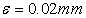. The network of conduits also includes diverse appurtenances simulating singular head losses. It includes a Venturi meter, an orifice meter, a 45° bend, a ball valve, a sudden enlargement, a sudden contraction, two 90° bends of large and small radius of curvature respectively, a strainer, a gate valve and an open valve.

Measurements are carried out in terms of height using water and mercury manometers. They represent the difference of pressure between the respective positions where they are realized. The necessary flow discharges are obtained through two centrifugal pumps, and the flow rate is insured by a rotameter.

### 3. Theoretical Approach

3.1. Frictional or Major Head Losses

Frictional head losses are mainly due to the fluid viscosity and the flow regime. Their influence may be resented throughout the length of the pipe. In a long pipe, the frictional head losses are relatively important, and they cannot be neglected. A relationship expressing this loss is proposed by . Known as the Darcy-Weisbach equation, it links the head loss the friction coefficient, the flow velocity and the pipe dimensions: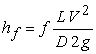(1)

By expressing the velocity as a function of flow pipe section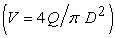and by replacing the known parameters by their respective numerous values, equation (1) becomes: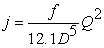(2)

Dimensional analysis of the Darcy-Weisbach equation leads to: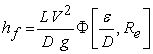(3)

Comparing equations (1) and (3) shows that the Darcy friction coefficient f is a function of both relative roughness and Reynolds number.  led the way in trying to express the friction coefficient by carrying out extensive experimentations leading to the measurement of the velocity distribution and head losses throughout the length of smooth and artificially roughened pipes. He applied the well-known Prandtl mixing length theory, and for smooth turbulent flow, developed a relationship for f which is unfortunately of implicit type requiring a recursive solution. For such a flow filed and for values of the Reynolds number less than 105,  reached a much more simple relation: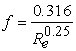(4)

However, almost all pipe flow regimes correspond to a transition zone where the frictional coefficient depends both on the Reynolds number and the relative roughness.  suggested a relationship in this direction since termed the Colebrook-White equation. It also had an implicit form, but was interesting since almost all the explicit solutions proposed later are approximations of this relation.  derived a complex relationship which has the advantage of being explicit and highly accurate for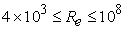and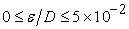: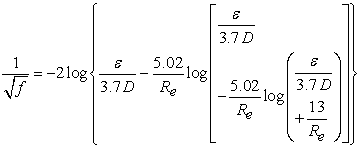(5)

Other empirical relationships proposed later have the merit of expressing the head loss without the complexity introduced by the friction coefficient. They are mostly based on polynomial representation. Mostly used in the U.S.A., the relationship developed by  may be written as: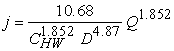(6)

The value of the Hazen-Williams coefficient CHW is a function of the material and its dimensions. Its value is found to be 145, 146, 148 and 150 for PVC-roughened-14 mm-diameter pipes, PVC-roughened-25 mm -diameter pipes, PVC-smooth-13.3 mm -diameter pipes, and PVC-smooth-23.5 mm -diameter pipes respectively .

Another often used relationship is that known as the Manning equation, although its attribution to this later is contested as reported by  and . It is expressed as: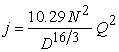(7)

The Manning roughness coefficient is solely dependant on the pipe material. Its value is found to be 0095. 0 for the type of PVC used in the experimentations .

The other relationships investigated in this study are those due to:

Strickler :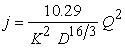(8)

Scobey, 1966: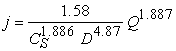(9)

Calmon and Lechapt :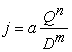(10)

where: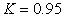for the type of pipes and fittings used.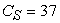for the new pipes used.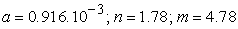for the smooth pipes used.for the roughned pipes used.

3.2. Singular Or Minor Head Losses

Head losses due to singularities or accessories are commonly termed minor head losses. This is due to the fact that for pipes of important length, their value can be neglected comparatively to that due to friction. However, their effect can be significant for short pipes. Minor head losses are expressed as: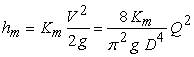(11)

The constant Km is a characteristic of the type of singularity, and its value varies consequently.

### 4. Results and Comparison

Figure 1 to Figure 4 present the results obtained concerning the frictional head losses for the four pipes investigated. They are presented in terms of the linear head loss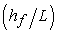versus the Reynolds number. All flow regimes were found to be turbulent.

For the two smooth pipes for which the results are presented in figure 1 and figure 2, the Hazen-Williams and Darcy approaches seem to be the more able to predicting the head loss. The integration of complex expressions for the friction coefficient with their large spectrum of application, like the one expressed in equation (5) , seems to be appropriate and ends up with satisfactory results.

It is nevertheless remarkable that the increase in the Reynolds number leads to higher errors. This is probably due to the fact that the flow field moves from the laminar/transitional regime to the turbulent regime. This difference can be corrected by integrating appropriate coefficients considering the change of regime, and this shows the complexity and the sensibility of the problem particularly when it is applied for the solution of distribution networks which may contain a great number of pipes with distinct diameters and roughness . This problem is not met when one deals with the energy losses encountered in pipelines transporting petroleum since these have usually the same characteristics .

The same comments can be made for the case of the roughened pipes for which the results are presented in figure 3 and figure 4. The experimental results show head losses greater than those computed by the different empirical formulas used. The authors comment on this difference by the fact that the roughness coefficient which is the most important parameter might have not been suitably predicted. Its value has in fact been chosen equal to that suggested by the manufacturer, thus neglecting the possible presence of deposits which generally tends to increase the roughness of pipe walls and consequently the head loss.Download asVeiw figureFigures index
Figure 1. Head loss for a smooth pipe (D=23.5 mm)Download asVeiw figureFigures index
Figure 2. Head loss for a smooth pipe (D=13.3 mm)Download asVeiw figureFigures index
Figure 3. Head loss for a roughened pipe (D=25 mm-ε = 0.015 mm)Download asVeiw figureFigures index
Figure 4. Head loss for a roughened pipe (D=14 mm-ε = 0.02 mm)

The capacity of transportation of pipelines decreases with time (age). This loss is mainly caused either by a diminution in the cross section area due to an accumulation of deposits inside the pipe, or an increase of the ruggedness, or both. The biological growth, the obstruction and the encrustation are the most common forms of such deposits which can vary from 1 mm to 10 mm in thickness. For the Hazen-Williams formula, the value of 140 is the most commonly used for CHW. It is however understood that for an old pipe in good condition, a value comprised in the interval 120 100 - should be acceptable, while for a used pipe 80 40 - is mostly used. This situation shows the great difficulties faced by the authors in achieving an ‘acceptable’ value for the coefficients (CHW as well as f and N) used in the developed relationships.

#### Table 1. Head loss for SingularitiesDownload asPowerPoint Slide

Veiw figure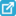View current table in a new window

The results obtained through the different singularities investigated are resumed in Table 1. Great difficulties have been faced by the authors when dealing with minor losses. Indeed, the measurements through such geometries have been found to be complex and, the literature dealing with such phenomenon is poor compared to that interested in the friction losses. In the case of a sudden enlargement for example, the re-establishment of the velocity field takes a distance of approximately 100 the pipe diameter. Within this intermediate region, the flow is complex involving both friction and turbulence, and it is difficult to separate the effects of the latter from that due to friction. Furthermore, the results published suggest values for the minor head loss coefficients generally neglecting important parameters such as material which may have a great influence upon the result.

The results proposed for the minor losses seem to be acceptable, except in the case of the sudden enlargement where there is a clear divergence. The authors feel that more interest should be given to this kind of local losses, and that future investigations should try to take into account all the factors involved in such head losses.

### 5. Conclusions

The present study investigated the different approaches developed for the determination of energy losses inside fluid transportation pipes. These losses may be caused either by friction or appurtenances.

The experimental results obtained and their comparison to those computed using the relationships developed by diverse investigators over the last decades show a preponderance of the relations proposed by Darcy and Hazen-Williams. The errors found seem to be mainly due to the difficulty to determining the friction coefficient which is a function of the Reynolds number and wall pipe roughness. The main difficulty which arises when trying to determine the pipe roughness is due to the fact that such pipes are subject to age effect resulting in erosion, corrosion, deposits, etc. The coefficient value proposed by the manufacturer is no more valid. Further research might well then be devoted to the phenomenon of aging of pipes.

More complexity is faced when head losses through singularities are investigated. The intermediate region downstream of any appurtenance is a mixture of friction and turbulence phenomena, and it is difficult to separate the effects of each one. Further research should be directed towards defining precise minor coefficients by taking into account all the factors involved in such head losses.

The results presented however do form a set of benchmark data for possible improvement and application in similar cases involving flow transportation inside pipes.

### Notations

a,m,n: Calmon-Lechapt coefficients

CHW: Hazen-Williams coefficients

CS: Scobey coefficient

D: Pipe diameter (m)

f: Pipe frictional coefficient

g: Gravitational acceleration (m/s2)

hf: Pipe frictional head loss (m)

hm: Pipe singular head loss (m)

j: Head loss per unit length (m/m)

K: Strickler coefficient

Ka: Karman number (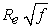)

Km: Singularity coefficient

L: Pipe length (m)

N: Manning roughness coefficient

Q: Fluid flow rate (m3/s)

  Barr, D.I.H., 1975, “New Forms of Equation for the correlation of Resistance Data”, Proc. Inst. of Civil Engrs., 59 (2), pp 827-835.In article View Article  Bhave, P.R., 1991, “Analysis of flow in water distribution networks”, Technomic Pub. Co., Inc., USA.In article  Blasius, H., 1913, “DasAhnlichkeitsgesetz in Flussigkeiten”, Verein Deutscher Ingenieure, Forschungsheft, p 131.In article  Boudebza C. and Bouachari, A., 1999, “Etude des approches de calcul des pertes de charge par frottement et singulières et comparaison avec l’expérimentation”, Guelma Univ., Algeria.In article  Chen J.J.J., 1984, “A Simple Explicit Formula for the Estimation of Pipe Friction Factor”, Proc. Inst. of Civil Engrs., 77 (2), pp 49-55.In article View Article  Chen, J.J.J., 1985, “Systematic Explicit Solutions of the Prandtl and Colebrook-White Equation for Pipe Flow”, Proc. Inst. of Civil Engrs., pp 383-389.In article View Article  Churchill, S.W., 1977, “Friction Factor Equation Spans All Fluid Flow Regimes”, Chemical Engineering, 84, pp 91-92.In article  Colebrook, C.F., 1939, “Turbulent Flow in Pipes with Particular reference to the Transition Region between the Smooth and Rough Pipe Laws”, J. Inst. of Civil Engrs., I1, pp 133-156.In article View Article  Ger, A.M. and Holly, E.R., 1976, “Comparison of single point injections in Pipe Flow”, J. Hydraul. Div., Am. Soc. of Civil Engrs., 102 (HY6), pp 731-746.In article  Haaland, S.E., 1983, “Simple and Explicit Formulas for the Friction Factor in Turbulent Pipe Flow”, J. Fluids Engrs., pp 89-90.In article View Article  Haddad, A., Bouachari, A. and Boudebza, C., 1999, “Pertes de charge régulières et singulières le long du tronçon Oléoduc SP-3”, Internal report, Guelma Univ., Algeria.In article  Haddad A. and Lahiouel Y., 1999, “Analyse des réseaux de distribution d’eau par la méthode de Cross et l’approche linéaire”, Trans. of the 4th Mech. Engg.Cong., vol. 2, p 89, Mohammadia, Morocco.In article  Jain, A.K., 1976, “Accurate Explicit Equation for Friction Factor”, J. Hydraul. Div., Am. Soc. of Civil Engrs., pp 674-677.In article  Jain, A.K., Mohan, D.M. and Khanna, P., 1978, “Modified Hazen-Williams Formula”, J.Environ. Engrg. Div., Am. Soc. of Civil Engrs., pp 137-146.In article  Johnson, S.P., 1934, “A Survey of Flow Calculation Methods”, Preprinted Paper for Summer Meeting, Am. Soc. of Mech. Engrs.In article  Lamont, P., 1969, “The Choice of the Pipe Flow Laws for Practical Use”, Water and Water Engineering, pp 55-63.In article  Moody, L.F., 1944, “Friction Factors for Pipe Flow”, Trans. American Society of Mechanical. Engineers., 66, pp 671-684.In article  Morel, M.A. and Laborde, J.P., 1994, “Exercices de Mécanique des Fluides”, Volume 1, Chihab-Eyrolles Editions, Algeria.In article  Nikuradse, J., 1933, “Strِmungsgesetze in Rauben Rohren”, Verein Deutsher Ingenieure, Forschungsheft, p 361.In article  Powell, R.W., 1968, “The Origin of Manning Formula”, J. Hydraul. Div., American Society of Civil Engrs., pp 1179-1181.In article  Rouse, H., 1943, “Evaluation of Boundary Roughness”, Proc. 2nd Hydraul. Conf., Univ. of Lowa.In article  Scobey, F.C., 1966, “The flow of water in commercially smooth pipes”, Water Ressources Center Archives, Series report 17, Univ. of California, Berkeley, USA.In article  Swamee, P.K. and Jain, A.K., 1976, “Explicit Equations for Pipe Flow Problems”, J.Hydraul. Div., Am. Soc. of Civil Engrs., 102 (HY11), pp 1707-1709.In article  Techo, R., Tichner, R.R. and James, R.E., 1965, “An Accurate Equation for the Computation of Friction Factor for Smooth Pipes from the Reynolds Number”, J. of Applied Mechanics, 32, pp 443.In article View Article  Weisbach J., 1855, “Die Experimental Hydraulik”, Freiberg, Germany: Engelhardt.In article  White, W.R., 1974, “The Hydraulic Characteristics of Clayware Pipes”, Hydraulic Research Station, Report No. Int. 133, Wallingford, Berkshire, England.In article  Williams, G.S. and Hazen, A., 1933, “Hydraulic tables”, 3rd Edition, John Wiley & Sons nc., USA.In article  Williams G.P., 1970, “Manning Formula-A Misnomer?”, J. Hydraul. Div., American Society of Civil Engrs., pp 193-200.In article  Wood, D.J., 1972, “An Explicit Friction Factor Relationship”, Civil Engineering, Am. Soc. of Civil Engrs. pp 383-390.In article PubMed  Zigrang, D.J. and Sylvester, N.D., 1982, “Explicit Approximations to the Solution of Colebrook’s Friction Factor Equation”, J. Am. Inst. of Chemical Engrs., pp 514-515.In article